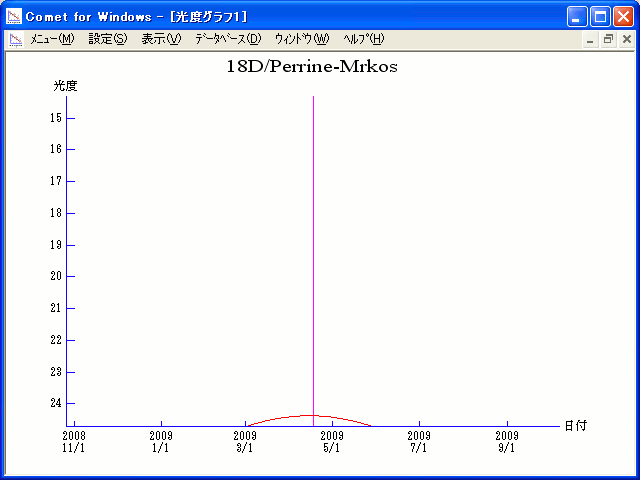# \$B%Q!<%i%\$%s(B-\$B%`%k%3%9WB@1(B

18D/Perrine-Mrkos (2009)###\$B50F;MWAG(B

```Perturbed ephemeris below is based on elements from NK 573.

Epoch = 2454920.50000 TT   T = 2454938.75931 TT
Peri. =  156.80416         a =     3.9442172 AU
Node  =  238.06666 J2000.0 e =     0.5851811
Incl. =   16.86718         q =     1.6361359 AU
```

###\$B8wEYJQ2=(B

```        m1 = 18.0 + 5 log\$B&\$(B + 20.0 log r
```##### \$B50F;MWAG\$O(BMinor Planet & Comet Ephemeris Service\$B\$G7W;;\$7\$?\$b\$N\$G\$9!#(B \$B8wEY%0%i%U\$O(BComet for Windows\$B\$G:n@.\$7\$?\$b\$N\$G\$9!#(B